# 2 2 Estimating Products by Rounding Objective SWBAT

• Slides: 102. 2 Estimating Products by Rounding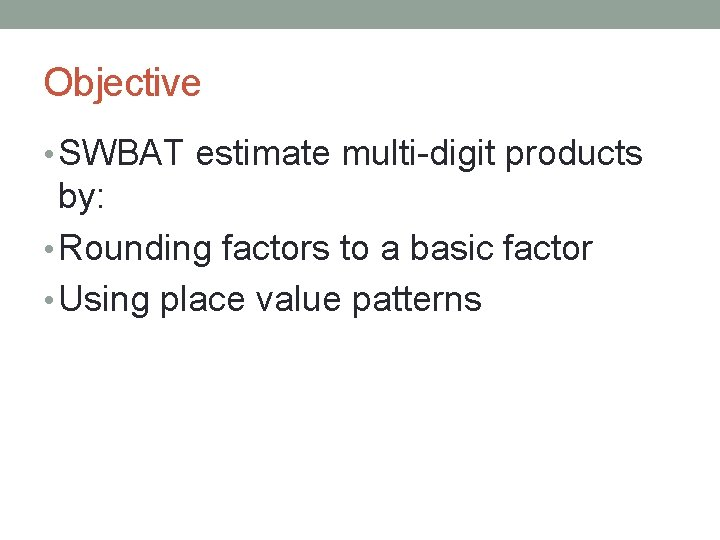Objective • SWBAT estimate multi-digit products by: • Rounding factors to a basic factor • Using place value patterns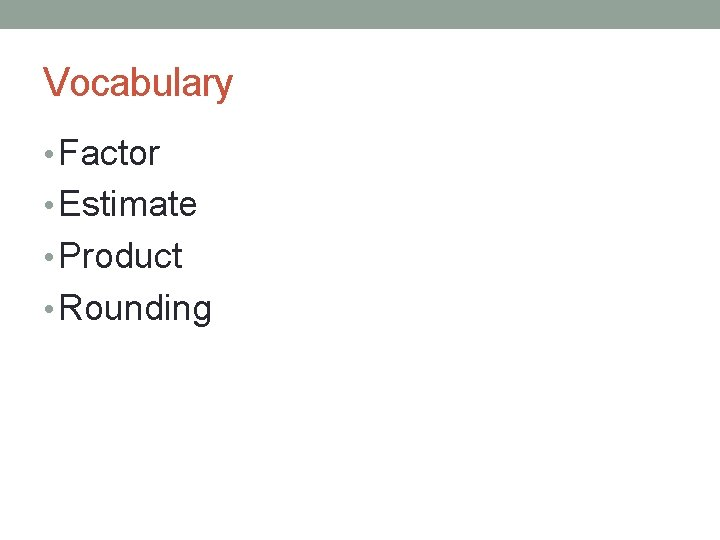Vocabulary • Factor • Estimate • Product • Rounding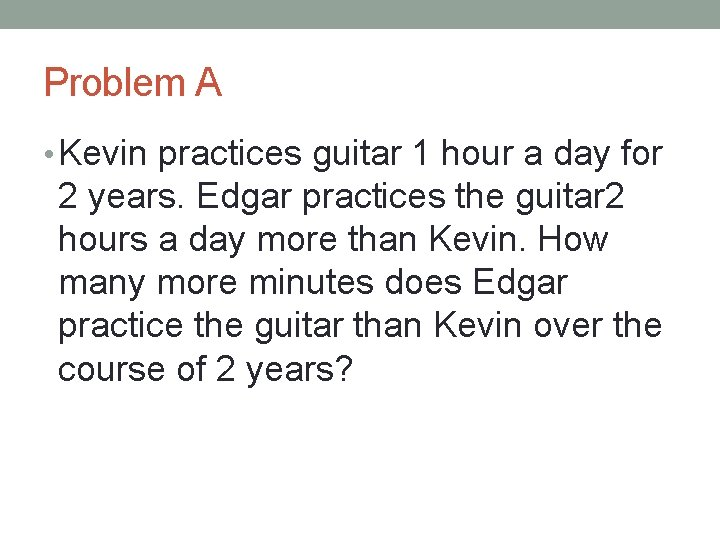Problem A • Kevin practices guitar 1 hour a day for 2 years. Edgar practices the guitar 2 hours a day more than Kevin. How many more minutes does Edgar practice the guitar than Kevin over the course of 2 years?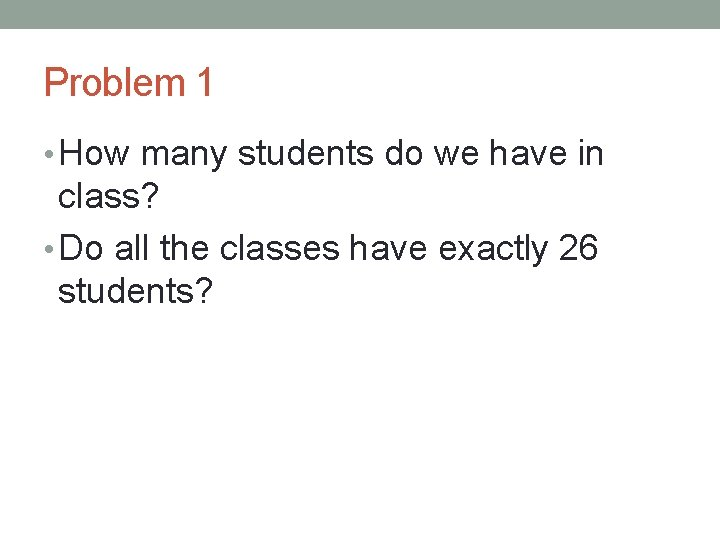Problem 1 • How many students do we have in class? • Do all the classes have exactly 26 students?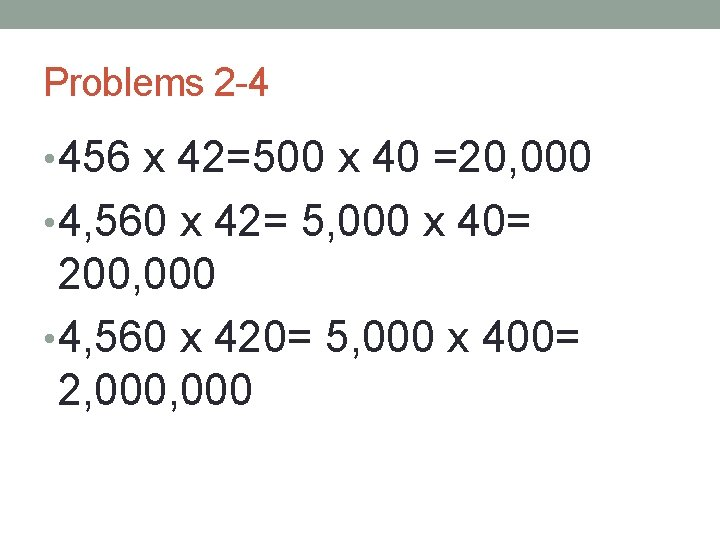Problems 2 -4 • 456 x 42=500 x 40 =20, 000 • 4, 560 x 42= 5, 000 x 40= 200, 000 • 4, 560 x 420= 5, 000 x 400= 2, 000Problems 5 -7 • 1, 320 x 88= 1, 000 x 90= 90, 000 • 13, 205 x 880= 10, 000 x 900= 9, 000 • 3, 120 x 880= 3, 000 x 900= 2, 700, 000 • 31, 200 x 880= 30, 000 x 900= 27, 000Problem Set 2. 2 • DO NOT WRITE ON THE PAPER!!! • Complete problem set 2. 2 by copying down the problems and solving them. • Once you are done, compare your answers with the person next to you. • You have 15 minutes to complete these problems. • After 15 minutes is up, we share our answers.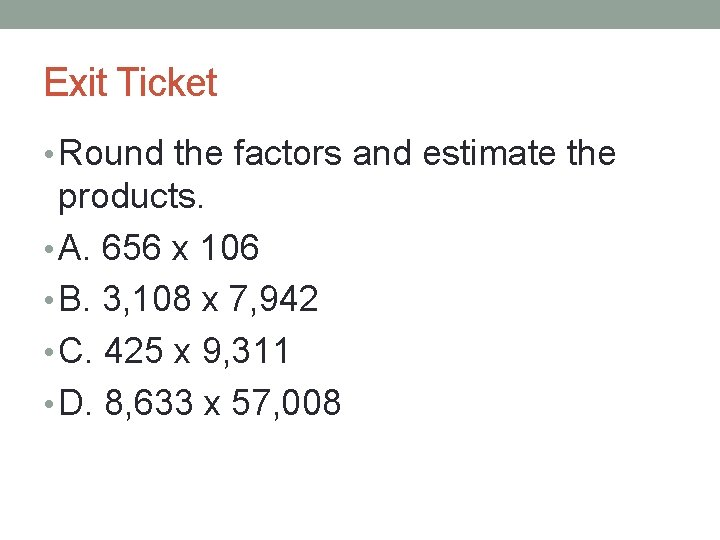Exit Ticket • Round the factors and estimate the products. • A. 656 x 106 • B. 3, 108 x 7, 942 • C. 425 x 9, 311 • D. 8, 633 x 57, 008Homework Complete the following problems by rounding the factors to estimate the products • 43 x 67 • 3, 938 x 436 • 1, 463 x 27 • 12 x 26 • 383 x 45 • 738 x 378 • 2, 890 x 55 • 8, 208 x 5, 132 • 733 x 562 • 57 x 89